# 3) Calculate volt) in terms of the given circuit parameters vitt Show your complete work here...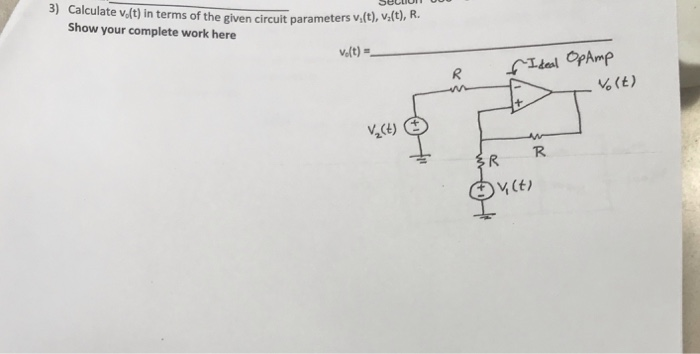3) Calculate volt) in terms of the given circuit parameters vitt Show your complete work here v,(t), v(t), R. Velt) %(E) R. V (t)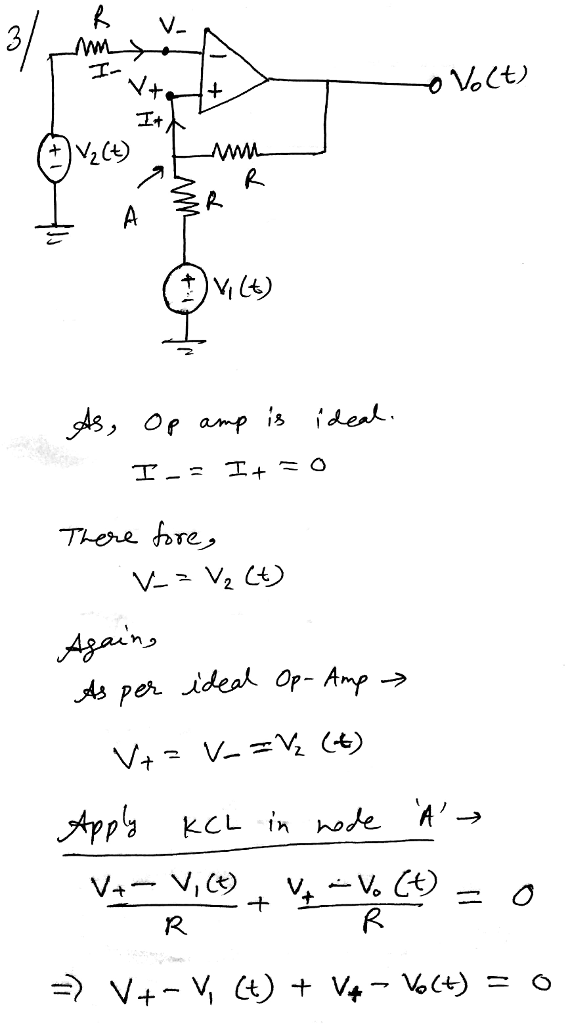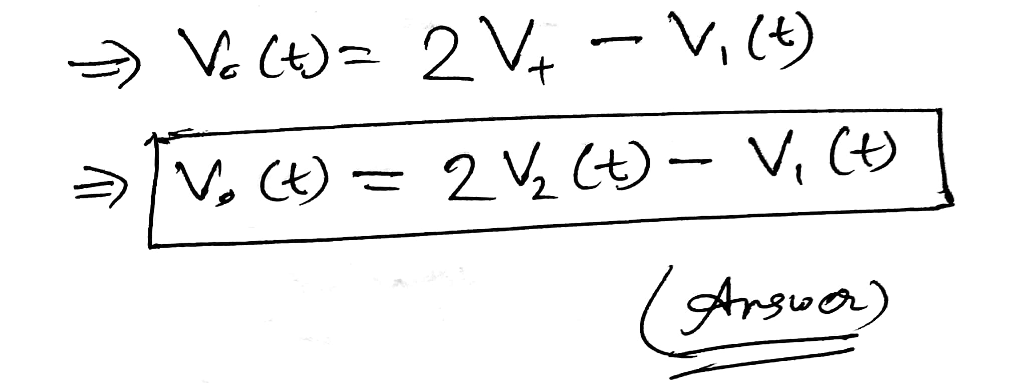#### Earn Coin

Coins can be redeemed for fabulous gifts.

Similar Homework Help Questions
• ### 4) Find the h parameters for the given circuit in terms of the “s” variable. Calculate...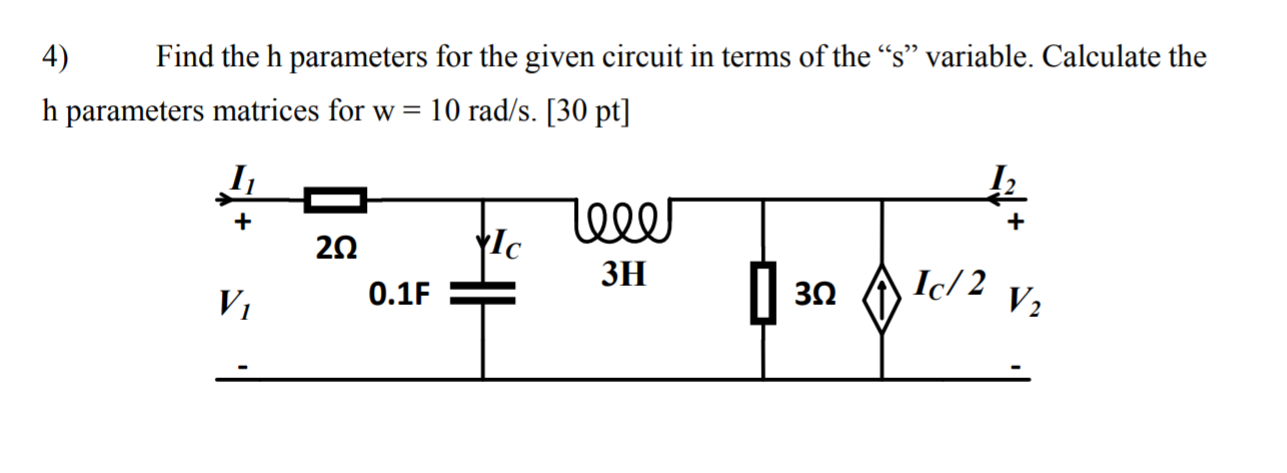4) Find the h parameters for the given circuit in terms of the “s” variable. Calculate the h parameters matrices for w= 10 rad/s. [30 pt] + 15 + Ylc 222 0.1F lel ЗН V 322 Ic/2 V2

• ### Include work. Calculate each of the circuit parameters for the provided circuit. RT(K) = Is(mA) =...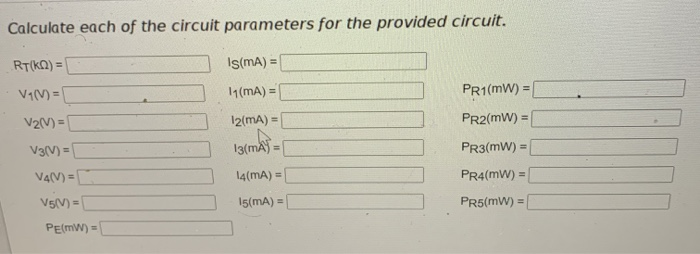Include work. Calculate each of the circuit parameters for the provided circuit. RT(K) = Is(mA) = V1(V) = PR1(MW) = V2(V) = PR2(mW) = 11(mA) = 12(mA) 13(mA) = 14(MA) = V3(V) = PR3(mW) = PR4(mW) = V4(V)= V5(V) = PE(MW) = 15(MA) = PR5(mW) = + V1 - 11 w + V3 - 13 R (50) R3 (30) 12 + E = 40V R2(10km) V2 R4(62) 314 14 Is Rs(1ko) Vs+

• ### Given the circuit below, determine the transfer function V (s)/V,(s) in terms of the parameters given....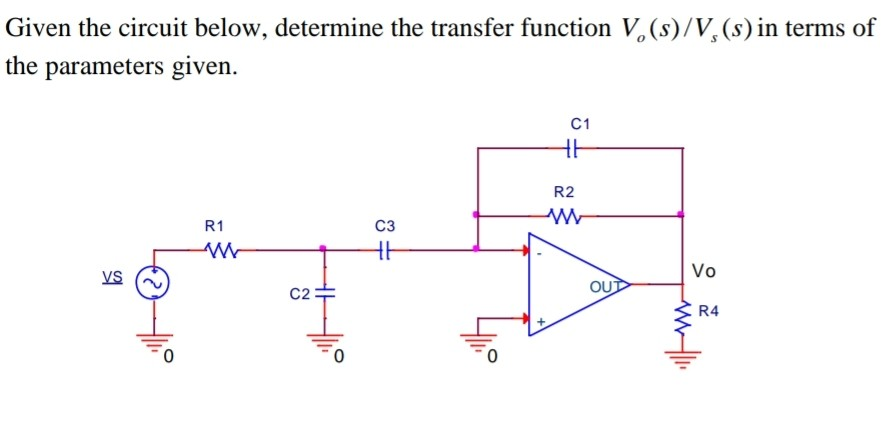Given the circuit below, determine the transfer function V (s)/V,(s) in terms of the parameters given. LE +? UD ok

• ### Chapter 7, Problem 7.113 X Incorrect. Find volt) for t>O in the circuit in the accompanying...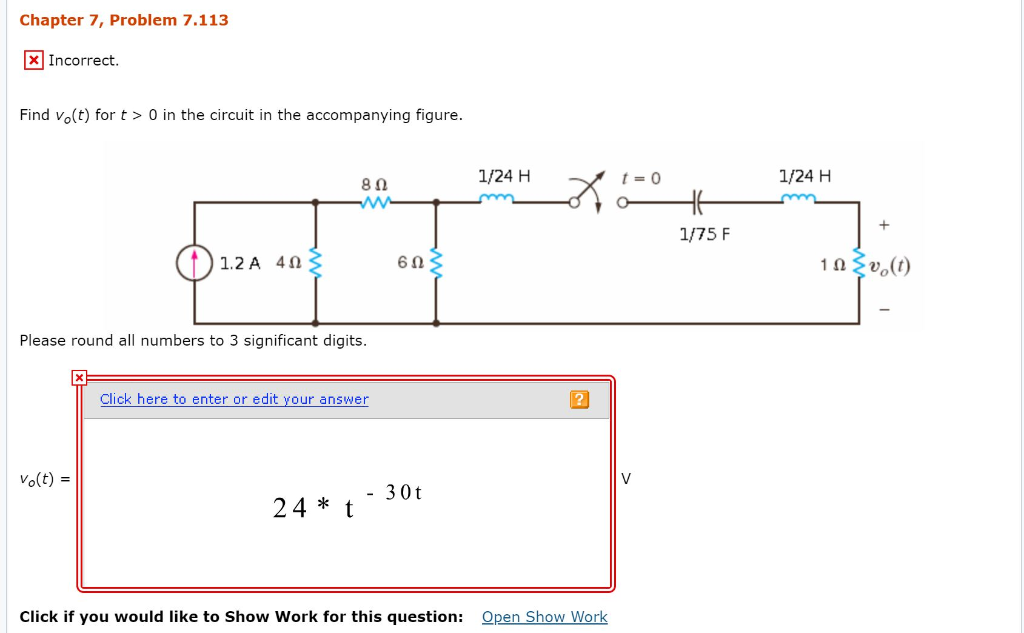Chapter 7, Problem 7.113 X Incorrect. Find volt) for t>O in the circuit in the accompanying figure. 80 1/24 H x t =0 1/24 H -Am 1/75 F 10 O 1.2A 40 60 v.(t) Please round all numbers to 3 significant digits. Click here to enter or edit your answer Volt) = - 30t 24 * t." Click if you would like to Show Work for this question: Open Show Work

• ### Given the parallel plate shown below (a) Calculate the TEM mode circuit parameters (L',C',R',and ...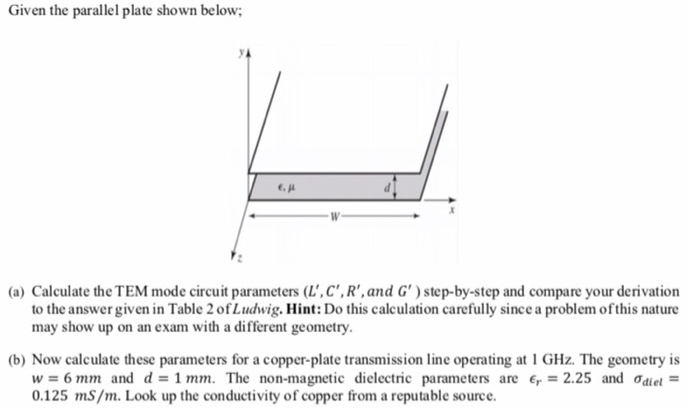Given the parallel plate shown below (a) Calculate the TEM mode circuit parameters (L',C',R',and G')step-by-step and compare your derivation to the answer given in Table 2 of Ludwig. Hint: Do this calculation carefully since a problem ofthis nature may show up on an exam with a different geometry. b) Now calculate these parameters for a copper-plate transmission line operating at 1 GHz. The geometry is w=6mm and d = 1 mm. The non-magnetic dielectric parameters are Er-2.25 and ơdiel 0.125...

• ### Fig. 5 shows a converter circuit with the following parameters: V = 110 V, L =...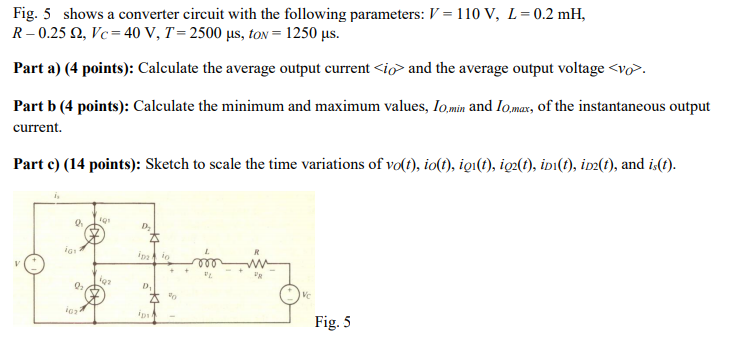Fig. 5 shows a converter circuit with the following parameters: V = 110 V, L = 0.2 mH, R-0.25 12, Vc=40 V, T= 2500 us, ton = 1250 us. Part a) (4 points): Calculate the average output current <io> and the average output voltage <vo. Part b (4 points): Calculate the minimum and maximum values, lo min and lomax, of the instantaneous output current. Parte) (14 points): Sketch to scale the time variations of volt), io(t), igi(t), ig2(t), iDi(t), ip(t),...

• ### 7.53 For the circuit shown in Fig. P7.53, draw a complete small-signal equivalent circuit utilizing an...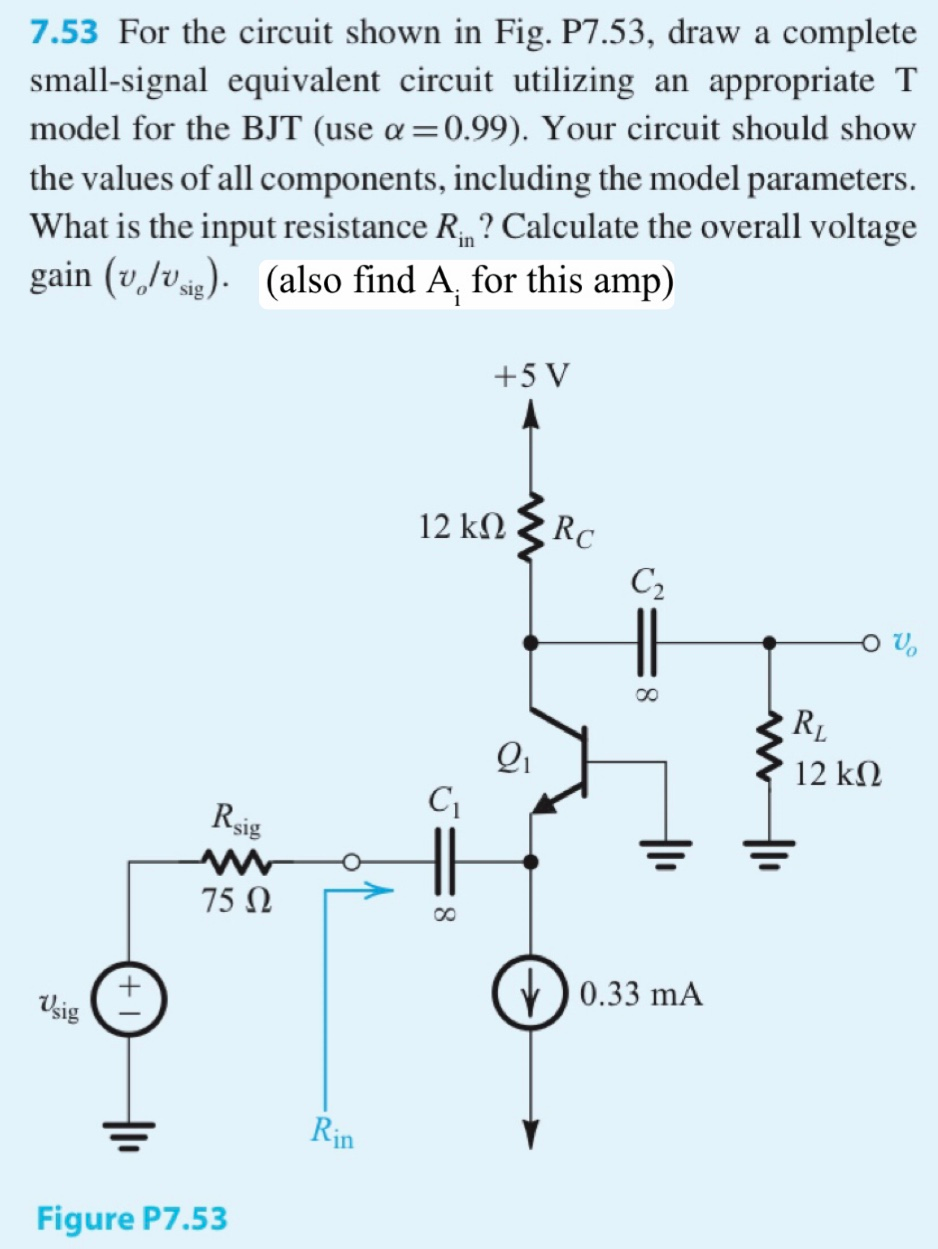7.53 For the circuit shown in Fig. P7.53, draw a complete small-signal equivalent circuit utilizing an appropriate T model for the BJT (use a =0.99). Your circuit should show the values of all components, including the model parameters. What is the input resistance R ? Calculate the overall voltage gain (v,/v). (also find A, for this amp) sig +5 V RC 12 kΩ C2 RL 12 ΚΩ Rsig 75 N ) 0.33 mA Vsig Rin Figure P7.53

• ### R wW L Consider the electric circuit in the figure above. This circuit is described by...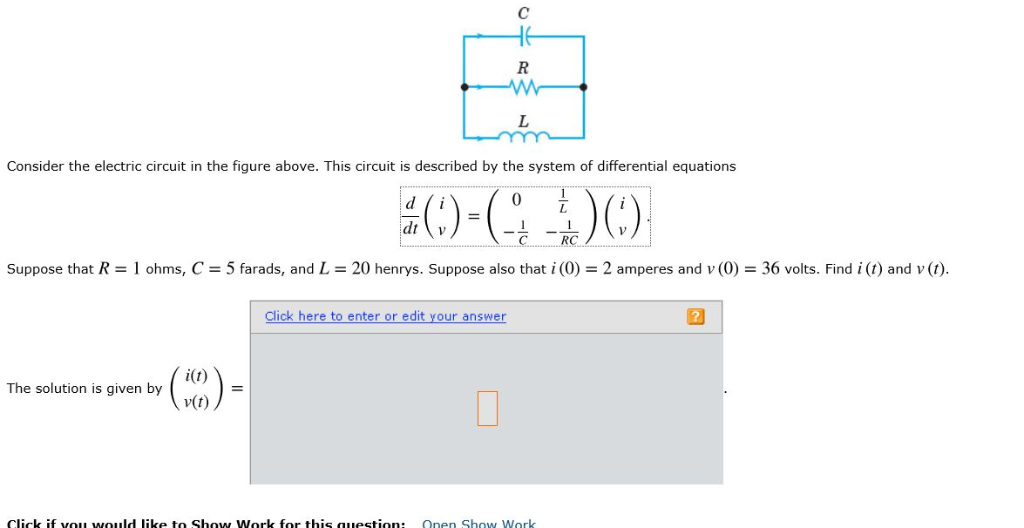R wW L Consider the electric circuit in the figure above. This circuit is described by the system of differential equations d dt RC Suppose that R = 1 ohms, C= 5 farads, and L 20 henrys. Suppose also that i (0) 2 amperes and v (0) 36 volts. Find i (t) and v (t). Click here to enter or edit your answer i(t) The solution is given by v(t) Click if vou would like to Show Work for this...

• ### Calculate each of the circuit parameters for the provided circuit. RT(N) = Is(mA) = V1(V) =...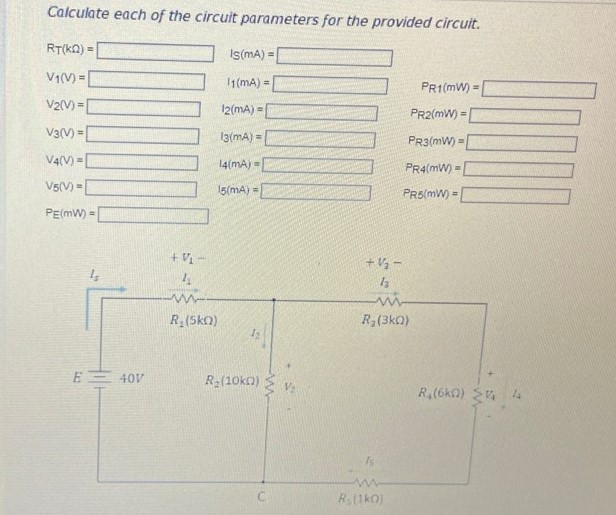Calculate each of the circuit parameters for the provided circuit. RT(N) = Is(mA) = V1(V) = 1(MA) = PR1MW) = V2(V) = 12(mA) = V3(V) = 13(mA) - PR2(MW) = PR3(MW) - PR4(MW) = 14(MA) - V4(V) - V5(V) = 15(mA) = PR5(W) - PE(MW) = + 1 + V3 - 13 W R (Sko) R (ko) E-40V Rz(2010) R (6) 4 W RO